# What is the kinematics of a particle

## Kinematics of the mass point

Subsections

Kinematics (E .: kinematics) deals with the description of the movement of a mass point (E .: mass point, point particle). Only the movement process is described without asking about its causes. That leads to the terms Location, speed and acceleration a mass point; the acceleration can naturally be broken down into two components, into which Tangential- and the Normal acceleration. For this description of the motion process, terms of differential geometry (curve theory) are required: The Arc length, the Tangential- and the Normal vector; the curvature or the Radius of curvature. These terms are explained in the 2nd paragraph.

In the course of the movement, the mass point describes a path (E .: trajectory) (Fig. 2.1). A coordinate system is introduced to describe them.

### location

The current position (position, E .: position) of the mass point is given by a vector from the coordinate origin (E .: origin) to the mass point.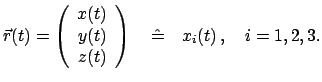(21)

Experience shows that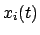are three continuous functions of time (vector function).

### speed

If one considers two (spatially and temporally) close positions of the mass point, then one obtains the speed (E .: velocity) from this by crossing the limit, see (Fig. 2.1).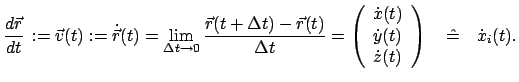(22)

Experience shows that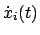are continuous functions of time. So you can specify the position and the speed of a mass point at any point in time. The absolute value of the speed is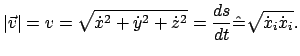(23)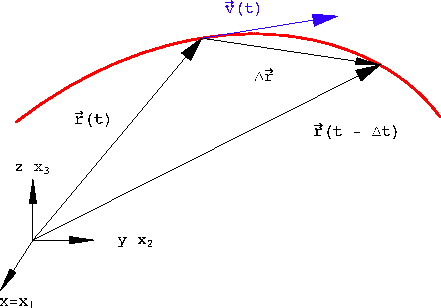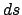is the differential of the arc length (see §2.2):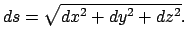(24)

Formula (2.3) can be interpreted physically: The amount of the speed is the length of the path covereddivided by the time interval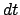that was needed to go through it.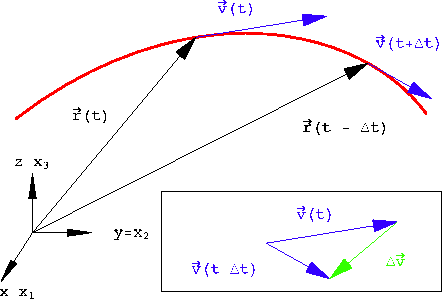### acceleration

The acceleration (E .: acceleration) is obtained again by crossing the limit if you consider two points that are close to each other and their speeds (Fig. 2.2).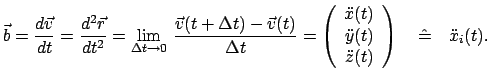(25)

Experience shows that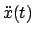are piecewise continuous functions of time. For example, there is a discontinuity in the acceleration when a point travels with a constant amount of speed on a curve that consists of a segment of a circular arc, which is followed by a straight stretch of path on both sides with a constant transition of the tangents. (see above 1 to chapter 2)

Summarizing the previous considerations, one can say: Theare piecewise twice continuously differentiable functions. One could define even higher derivatives; but these are generally not needed.

The acceleration can be broken down into two components, one in the direction of the velocity (Tangential acceleration), the other normal to speed (Normal acceleration) (Fig. 2.3).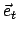or.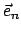are the unit vectors introduced in §2.2 in the tangential or normal direction (see Fig. 2.3).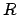is the radius of the circle of curvature. The formulas (2.6) to (2.8) are derived in the same way below. This derivation expresses the fact that a movement contains a geometric component (the shape of the trajectory) and a time sequence (on this trajectory). Differentiation according to the chain rule gives: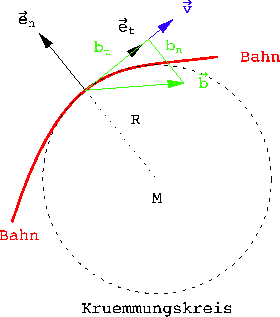These derivations can be summarized as follows: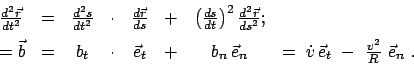(210)

For this transformation, the formulas (2.20) and (2.23) of the next paragraph were used:

The minus sign in the second formula occurs because the normal vectoris always directed outwards (i.e. away from the center of curvature, see Fig. 2.3).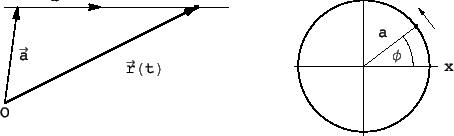Example 2.1: Any accelerated movement on a straight line (Fig.2.4 (a)):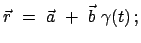(211)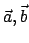are constant vectors;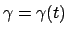a scalar, twice continuously differentiable function of time t. By calculation it is shown that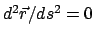: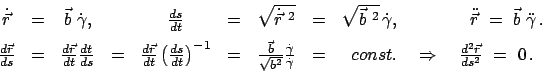Example 2.2: A point moves with constant angular velocity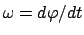on a circle of radius a (Fig.2.4 (b)). Show that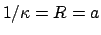.

For the second derivative of the position vector according to the length of the arc, the following results are obtained through repeated application of the chain rule: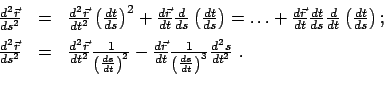(213)

Inserting the expressions calculated above into the above equation gives: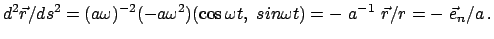(214)

When discussing the properties of the trajectory of a mass point, two groups of properties are distinguished. Some are related to the geometric shape of the trajectory; the others concern the course of the movement on this trajectory. The theory for describing the geometric properties of the trajectory is differential geometry. Some of the terms of these are to be presented in this section.

### Arc length

The arc length (E .: arclength) is required to determine the length of a curve segment. The most practical form of displaying a curve is in parameter form: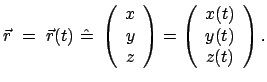(215)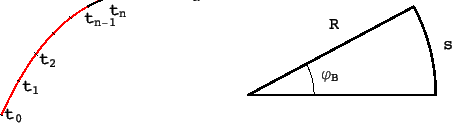An example of such a space curve is the helix (E .: screw, helix)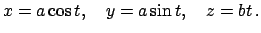(216)

The parameter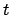can be proportional to time; but it could also represent a completely different quantity, such as an angle.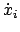if the derivation is according to this parameter, there is therefore generally no speed. The curve piece is divided into small parts (see Fig. 2.5 (a)). Each of these arc pieces is approximated by a straight line (Pythagorean theorem)

The mean theorem of differential calculus is applied to each of the differences.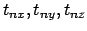are out of the interval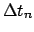. This is the route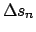: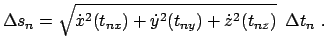The length of each section is allowed to approach zero. This border crossing has the effect that each of these sections becomes the differential of the arc length: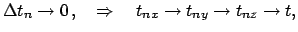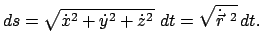(218)

It must be assumed that the curve is `` sufficiently smooth '' so that this limit crossing is permissible and sensible. For example, it can be assumed that the first derivatives that appear exist and are continuous almost everywhere in the areas under consideration. Counter-examples where this border crossing is not possible are the fractals.

According to (2.18), the arc length is the integral: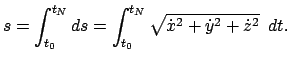(219)

An example is the determination of the length of an arc (Fig.2.5 (b)):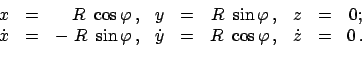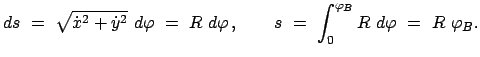### Tangent and normal vector

If you only want to investigate the geometrical shape of a space curve, you have to run through it with speed 1. To do this, you need the arc length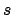as a parameter. Then the tangent vector (E .: tangent)everywhere the length 1. The conversion from the parameteron the arc lengthtakes place using the chain rule of differentiation and the expression (2.18) for the differential of the arc length. The normalized tangent vector is then: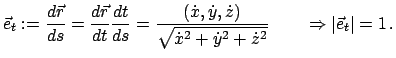(220)

The normal vector (E .: normal)is a unit vector that is perpendicular to the tangent vector and points to the outside of the curve (see Fig. 2.3). First, two properties of the second derivative of the position vector with respect to the arc length are proven:

1) The 2nd derivative is perpendicular to the first (= the tangent unit vector)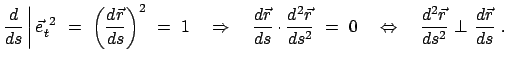(221)

2) The second derivative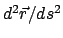is zero if and only if the curve is a straight line: a) According to the assumption, the curve is a straight line:

b) Conversely, it follows from the disappearance of the 2nd derivative: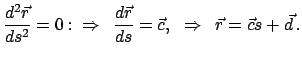i.e. the path is a straight line.

In Eq. (2.14) it is shown that when a point revolves on a circular path of radius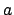applies: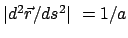.

The considerations at the end of the previous paragraph suggest using the absolute value of this 2nd derivative as a measure of the curvature (E .: curvature)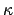to choose and define a curve: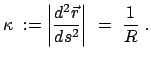(222)

R is called the radius of curvature. The curve is a circle of radius, then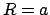. Summarizing (2.21) and (2.22), one can define: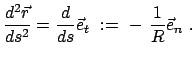(223)

The negative sign takes into account that the normal vector always points to the outside of the curve. The ones from the vectorsandThe spanned plane is called an oscillating plane. If you draw the center of the associated circle of curvature for each point of a given curve, you generally get another curve, the evolute. We will only need these once, namely with the Cycloid Pendulum, §6.2.1, and Notebook K6ZykloidenPend.nb.

The above formula (2.22) for the curvature can also be given a clearer form. For this purpose, in Eq. (2.13)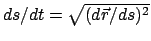used. In order to reduce the writing effort, the following abbreviations are used. Points mean derivatives according to the parameter, Dashes derivatives according to the arc length: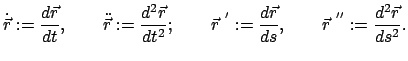For the curvature, the square of the final formula above is calculated:

If there is a plane curve in parametric representation, then the position vector and its derivatives have only two components. In this case, this formula for the square of the curvature simplifies to: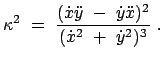(225)

The curvature of a scalar function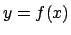can be found from the previous one using the approach: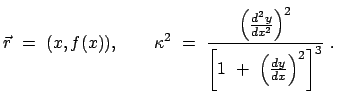(226)

Literature on §2.2:
IN. Bronstein, K.A. Semendjajew: Taschenbuch der Mathematik, Part III Analytical Geometry and Differential Geometry, II Differential Geometry.

Christian Sommer 2003-01-27